Home > INT1 > Chapter Ch5 > Lesson 5.2.2 > Problem5-68

5-68.
1. Determine the common difference (sequence generator) for each arithmetic sequence listed below. Then write an equation for the nth term in each sequence, keeping in mind that the first term of each sequence is t(1). Homework Help ✎

1. 4, 7, 10, 13, …

2. 3, 8, 13, …

3. 24, 19, 14, …

4. 7, 9.5, 12, …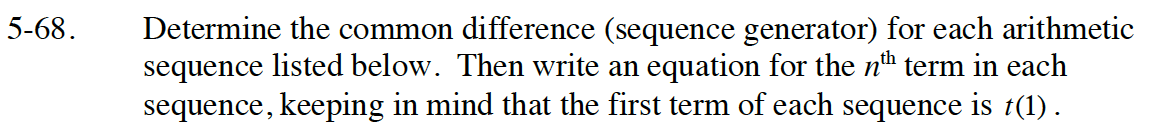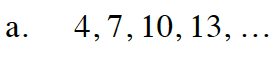m = 3

7 − 4 = 10 − 7 = 13 − 10 = 3

t(n) = 3n + t(0)
t(1) = 3(1) + t(0) = 4
3 + t(0) = 4
t(0) = 1

t(n) = 3n + 1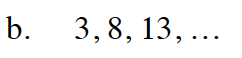m = 5

t(n) = 5n − 2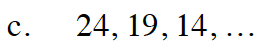t(n) = −5n + 29

m = −5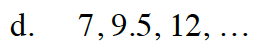9.5 − 7 = 12 − 9.5 = 2.5

m = 2.5

t(0) = 4.5

t(n) = 2.5n + 4.5